# Python For GCSE Section A Exercises

This is a screenshot of a simple program that adds up two numbers entered by the user. Type it up carefully, save the file and run it to test that it works as you expect.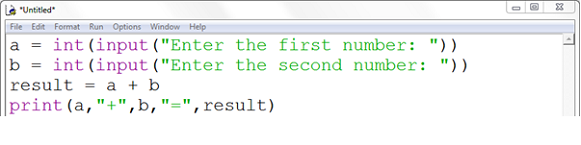Adapt the program so that, instead of adding, it subtracts the second number from the first. The picture below uses red splodges in the places that you need to change.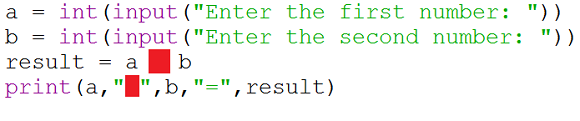Adapt the program so that, instead of adding, it multiplies the numbers together. The picture below uses red splodges in the places that you need to change.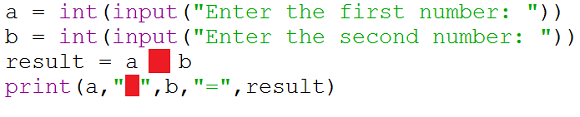Adapt the program so that, instead of adding, it divides the first number by the second. The picture below uses red splodges in the places that you need to change.Write a program that allows the user to input the radius of a circle and get the area and circumference. (PI = 3.14159, area = PI x R x R, circumference = 2 x PI x R). Replace the red sections of the following with the statements that are needed,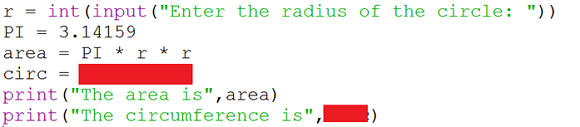A class has four exams in one term. Write a program that reads in these scores and returns the student's mean average mark.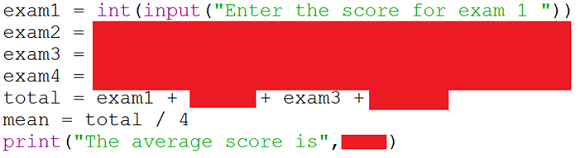A temperature in degrees Celsius can be converted to degrees Fahrenheit using the formula, F = (9/5) * C + 32. Write a program that allows the user to input a temperature in Celsius and output the equivalent in degrees Fahrenheit.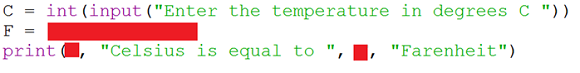Write a program where the user enters the length (L) and height (H) of a rectangle.

The perimeter (P) is equal to L+L+H+H

The area (A) is equal to L*H

Print each result on a separate line, like Task 4.

Write a program that gets the user to enter the base and height of a triangle. The program will output the area of the triangle.

Write a program that gets the user to enter a journey distance and the average speed of the car making that journey. The program should output the time taken for the journey in minutes.

Write a program that takes the three inputs required to calculate the area of a trapezium and outputs the area.

Write a program that calculates the volume of a cylinder. You will need the user to enter the radius of the cylinder's base and its height. The volume is the area of the base multiplied by the height.

Write a program that uses Pythagoras' Theorem to calculate the length of the longest side of a right angled triangle given the lengths of the shorter sides.

Write a program which allows the user to enter an amount of money as a whole number. Work out how to make this quantity in £20, £10, £5 notes and £2 and £1 coins.

Write a program that uses floor division and modulus operators to convert a number of minutes into minutes and seconds.

Write a program to simulate the rolling of two dice.

Write a program to calculate the volume and surface area of a sphere with a radius specified by the user. You will need to look up the formulas for these calculations.

Write a program to compute the distance between 2 points on a graph - the points (x1, y1) and (x2, y2). Use 4 input statements to gather the information from the user.

Write a program that accepts 3 integers from the user. Your program should output the integers from smallest to largest.

You cannot use any loops or conditional statements to do this. That would be too easy.

smallest = min(a,b,c)
largest = max(a,b,c)

The trick is to work out how to get the middle number. If you add the 3 numbers together, you will get the total of all 3. Since your program will know the largest and smallest (from the statements above), it shouldn't be hard to work out the middle number from that.

Write a program to work out the midpoint of a line defined by the points (x1, y1) and (x2, y2). Use 4 input statements to gather the information from the user.

Copy and test the following program. Look carefully at how the variables are used along with text to create a story.

```name = input("Enter a name: ")
animal = input("Enter the name of an animal: ")
superhero= input("Enter a superhero name: ")
superpower = input("Enter a superpower: ")

print(name, " was bitten by a radioactive ",animal, " and became ",superhero,".", sep="")
print(superhero, " had the ability to ",superpower, ".", sep="")
```

You are going to make your own version. Start by typing a short paragraph of text to finish off the story below. As you write, choose some words that can be replaced with choices made by the user. Make those words bold as I have below. You should have a story with 10 different words that the user enters.

Once there was a description boy called name. One adjective day, he was bitten by a radioactive animal.